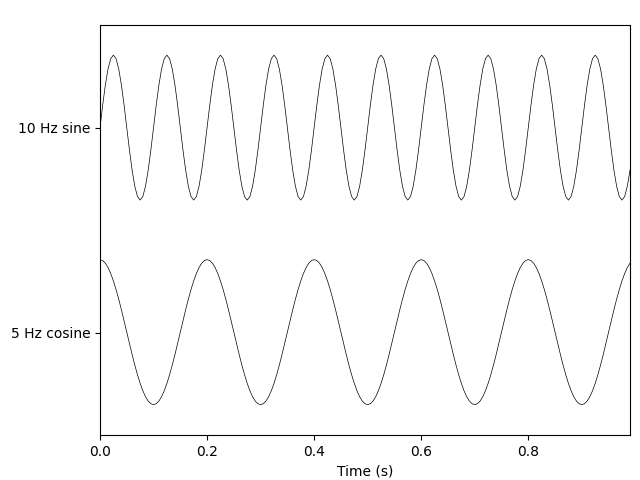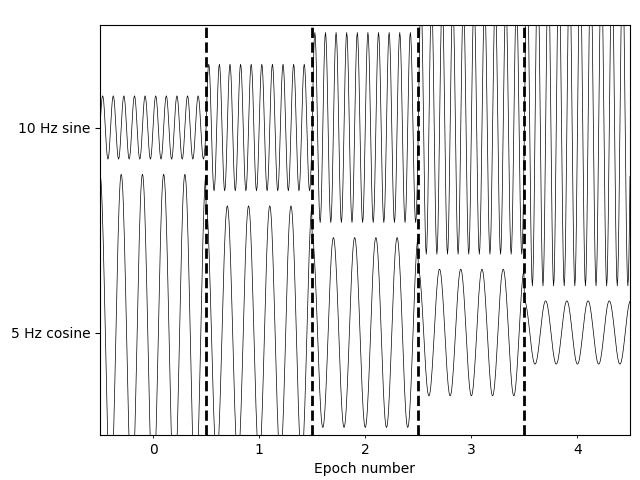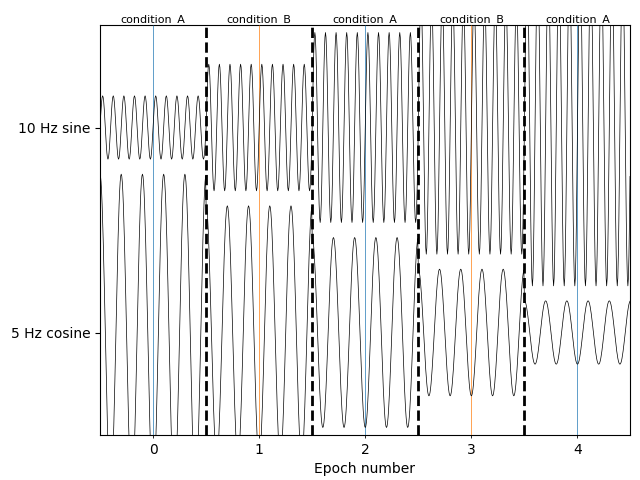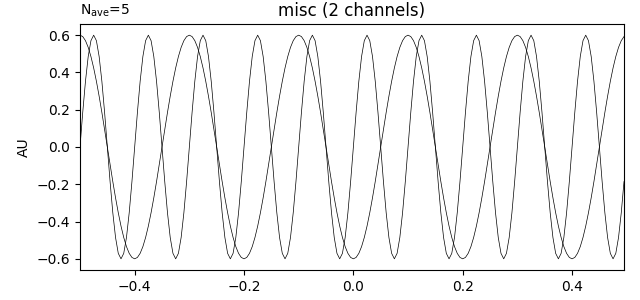Creating MNE-Python data structures from scratch¶

This tutorial shows how to create MNE-Python’s core data structures using an existing NumPy array of (real or synthetic) data.

We begin by importing the necessary Python modules:

import mne
import numpy as np

Creating Info objects¶

The core data structures for continuous (Raw), discontinuous (Epochs), and averaged (Evoked) data all have an info attribute comprising an mne.Info object. When reading recorded data using one of the functions in the mne.io submodule, Info objects are created and populated automatically. But if we want to create a Raw, Epochs, or Evoked object from scratch, we need to create an appropriate Info object as well. The easiest way to do this is with the mne.create_info function to initialize the required info fields. Additional fields can be assigned later as one would with a regular dictionary.

To initialize a minimal Info object requires a list of channel names, and the sampling frequency. As a convenience for simulated data, channel names can be provided as a single integer, and the names will be automatically created as sequential integers (starting with 0):

n_channels = 32
sampling_freq = 200  # in Hertz
info = mne.create_info(n_channels, sfreq=sampling_freq)
print(info)

Out:

<Info | 7 non-empty values
ch_names: 0, 1, 2, 3, 4, 5, 6, 7, 8, 9, 10, 11, 12, 13, 14, 15, 16, 17, ...
chs: 32 MISC
custom_ref_applied: False
highpass: 0.0 Hz
lowpass: 100.0 Hz
meas_date: unspecified
nchan: 32
projs: []
sfreq: 200.0 Hz
>

You can see in the output above that, by default, the channels are assigned as type “misc” (where it says chs: 32 MISC). You can assign the channel type when initializing the Info object if you want:

ch_names = [f'MEG{n:03}' for n in range(1, 10)] + ['EOG001']
info = mne.create_info(ch_names, ch_types=ch_types, sfreq=sampling_freq)
print(info)

Out:

<Info | 7 non-empty values
ch_names: MEG001, MEG002, MEG003, MEG004, MEG005, MEG006, MEG007, MEG008, ...
chs: 3 MAG, 6 GRAD, 1 EOG
custom_ref_applied: False
highpass: 0.0 Hz
lowpass: 100.0 Hz
meas_date: unspecified
nchan: 10
projs: []
sfreq: 200.0 Hz
>

If the channel names follow one of the standard montage naming schemes, their spatial locations can be automatically added using the set_montage method:

ch_names = ['Fp1', 'Fp2', 'Fz', 'Cz', 'Pz', 'O1', 'O2']
ch_types = ['eeg'] * 7
info = mne.create_info(ch_names, ch_types=ch_types, sfreq=sampling_freq)
info.set_montage('standard_1020')
Measurement date Unknown Unknown Unknown 10 points 0 magnetometer, 0 gradiometer, and 7 EEG channels Not available Not available 200.00 Hz 0.00 Hz 100.00 Hz

Note the new field dig that includes our seven channel locations as well as theoretical values for the three cardinal scalp landmarks.

Additional fields can be added in the same way that Python dictionaries are modified, using square-bracket key assignment:

info['description'] = 'My custom dataset'
print(info)

Out:

<Info | 10 non-empty values
ch_names: Fp1, Fp2, Fz, Cz, Pz, O1, O2
chs: 7 EEG
custom_ref_applied: False
description: My custom dataset
dig: 10 items (3 Cardinal, 7 EEG)
highpass: 0.0 Hz
lowpass: 100.0 Hz
meas_date: unspecified
nchan: 7
projs: []
sfreq: 200.0 Hz
>

Creating Raw objects¶

To create a Raw object from scratch, you can use the mne.io.RawArray class constructor, which takes an Info object and a NumPy array of shape (n_channels, n_samples). Here, we’ll create some sinusoidal data and plot it:

times = np.linspace(0, 1, sampling_freq, endpoint=False)
sine = np.sin(20 * np.pi * times)
cosine = np.cos(10 * np.pi * times)
data = np.array([sine, cosine])

info = mne.create_info(ch_names=['10 Hz sine', '5 Hz cosine'],
ch_types=['misc'] * 2,
sfreq=sampling_freq)

simulated_raw = mne.io.RawArray(data, info)
simulated_raw.plot(show_scrollbars=False, show_scalebars=False)Out:

Creating RawArray with float64 data, n_channels=2, n_times=200
Range : 0 ... 199 =      0.000 ...     0.995 secs

Creating Epochs objects¶

To create an Epochs object from scratch, you can use the mne.EpochsArray class constructor, which takes an Info object and a NumPy array of shape (n_epochs, n_channels, n_samples). Here we’ll create 5 epochs of our 2-channel data, and plot it. Notice that we have to pass picks='misc' to the plot method, because by default it only plots data channels.

data = np.array([[0.2 * sine, 1.0 * cosine],
[0.4 * sine, 0.8 * cosine],
[0.6 * sine, 0.6 * cosine],
[0.8 * sine, 0.4 * cosine],
[1.0 * sine, 0.2 * cosine]])

simulated_epochs = mne.EpochsArray(data, info)
simulated_epochs.plot(picks='misc', show_scrollbars=False)Out:

5 matching events found
No baseline correction applied
0 projection items activated

Since we did not supply an events array, the EpochsArray constructor automatically created one for us, with all epochs having the same event number:

Out:

[1 1 1 1 1]

If we want to simulate having different experimental conditions, we can pass an event array (and an event ID dictionary) to the constructor. Since our epochs are 1 second long and have 200 samples/second, we’ll put our events spaced 200 samples apart, and pass tmin=-0.5, so that the events land in the middle of each epoch (the events are always placed at time=0 in each epoch).

events = np.column_stack((np.arange(0, 1000, sampling_freq),
np.zeros(5, dtype=int),
np.array([1, 2, 1, 2, 1])))
event_dict = dict(condition_A=1, condition_B=2)
simulated_epochs = mne.EpochsArray(data, info, tmin=-0.5, events=events,
event_id=event_dict)
simulated_epochs.plot(picks='misc', show_scrollbars=False, events=events,
event_id=event_dict)Out:

5 matching events found
No baseline correction applied
0 projection items activated

You could also create simulated epochs by using the normal Epochs (not EpochsArray) constructor on the simulated RawArray object, by creating an events array (e.g., using mne.make_fixed_length_events) and extracting epochs around those events.

Creating Evoked Objects¶

If you already have data that was averaged across trials, you can use it to create an Evoked object using the EvokedArray class constructor. It requires an Info object and a data array of shape (n_channels, n_times), and has an optional tmin parameter like EpochsArray does. It also has a parameter nave indicating how many trials were averaged together, and a comment parameter useful for keeping track of experimental conditions, etc. Here we’ll do the averaging on our NumPy array and use the resulting averaged data to make our Evoked.

# Create the Evoked object
evoked_array = mne.EvokedArray(data.mean(axis=0), info, tmin=-0.5,
nave=data.shape, comment='simulated')
print(evoked_array)
evoked_array.plot()Out:

<Evoked | 'simulated' (average, N=5), -0.5 – 0.495 sec, baseline off, 2 ch, ~10 kB>

Total running time of the script: ( 0 minutes 17.487 seconds)

Estimated memory usage: 8 MB

Gallery generated by Sphinx-Gallery